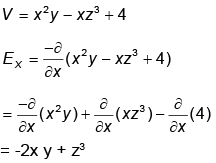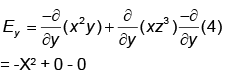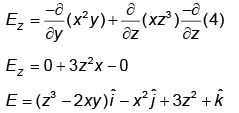Courses

# Electric Potential MCQ (With Solution) : Test 2

## 25 Questions MCQ Test Exclusive Video Lectures of Class 12 Physics by Experts | Electric Potential MCQ (With Solution) : Test 2

Description
This mock test of Electric Potential MCQ (With Solution) : Test 2 for Class 12 helps you for every Class 12 entrance exam. This contains 25 Multiple Choice Questions for Class 12 Electric Potential MCQ (With Solution) : Test 2 (mcq) to study with solutions a complete question bank. The solved questions answers in this Electric Potential MCQ (With Solution) : Test 2 quiz give you a good mix of easy questions and tough questions. Class 12 students definitely take this Electric Potential MCQ (With Solution) : Test 2 exercise for a better result in the exam. You can find other Electric Potential MCQ (With Solution) : Test 2 extra questions, long questions & short questions for Class 12 on EduRev as well by searching above.
QUESTION: 1

### An electric field is expressed as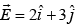. Find the potential diference (VA-VB) between two points A and B whose position vectors are given by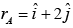and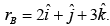Solution: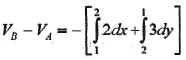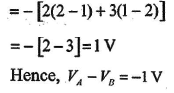QUESTION: 2

### The potential function of an electrostatic field is given by V = 2x2. Determine the electric field strength at the point (2 m, 0.3 m).

Solution: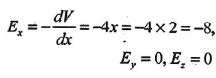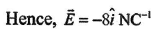QUESTION: 3

### Figure 3.79 shows equipotential surfaces concentric at O, the magnitude of electric field at a distance r measured from O is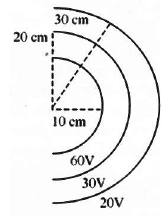Solution: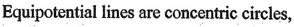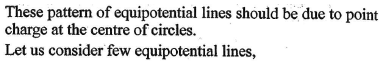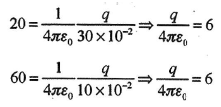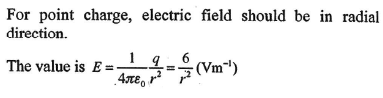QUESTION: 4

The electric field in a certain region is A/x3. Then the potential at a point (x,y, z), assuming the potential at infinity to be zero, is

Solution: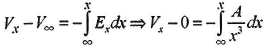⇒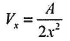QUESTION: 5

Find the potential V of an electrostatic field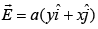, where a is constant.

Solution: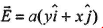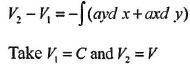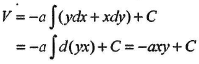QUESTION: 6

Consider a system of three charge q/3,q/3 and -2q/3 placed at points A,B and C, respectively, as shown in figure. Take O to be the circle of radius R and angle CAB = 60°.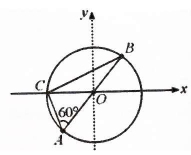Solution: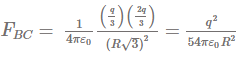QUESTION: 7

Two equal positive charges are kept at points A and B.The electric potential at the points between A and B (excluding these points) is studied while moving from A to B. The potential

Solution:

If we draw a graph of v - r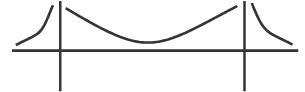We can clearly see that V first decreases and then increases.

QUESTION: 8

Figure shows three paths along which we can move positively charged sphere A closer to positively charged sphere B which is fixed in a plane. if W1, W2 and W3 are the work done along the three respective paths, then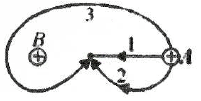Solution:

As work done is path independent due to conservative nature of electric field. W1 = W2 = W3

QUESTION: 9

The work done in moving an electron of charge e and mass m from A to B along the circular path (shown in figure) in the vertical plane in the field of charge Q is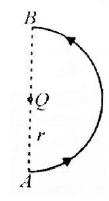Solution:

Work done by electric field will be zero as the semicircle will be equipotential, but we will have to consider the work done against gravity.
F = mg       W = F 2r = 2 mgr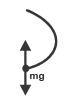QUESTION: 10

Four similar point charges q are located at the vertices of a tetrahedron with an edge a. The energy of the interaction of charges is :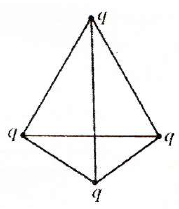Solution:

There are 6 pairs at gap a each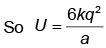QUESTION: 11

In the electric field of a point charge q, a certain charge is carried from point A to B, C, D and E. Then the work done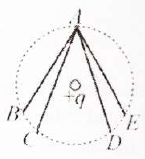Solution:

The circle will be equipotential so work done  will be zero for all paths.

QUESTION: 12

Four equal charges Q are placed at the four corners of a square of each side is a. Work done in removing a charge -Q from its centre of infinity is

Solution: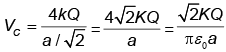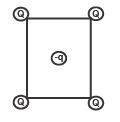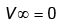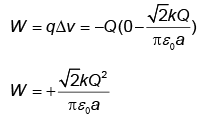QUESTION: 13

Ten electrons are equally spaced and fixed around a circle of radius R. Relative to V = 0 at infinity, the electrostatic potential V and the electric field E at the centre C are

Solution:

V is scalar so it will just get added up so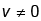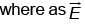is vector and its vecter sum will be zero.

QUESTION: 14

The displacement of a charge Q in the electric field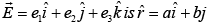. The work done is

Solution: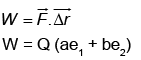QUESTION: 15

Three charges Q + q and +q are placed at the vertices of a right-angled isosceles triangle as shown. The net electrostatic energy of the configuration is zero if Q is equal to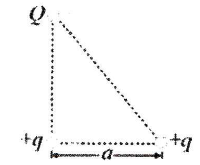Solution: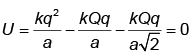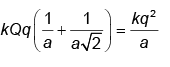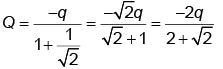QUESTION: 16

Electric potential at any point is V = - 5x +3y + √15z, then the magnitude of the electric field is

Solution:

V = -5x + 3y + √15z (in question is only over 15,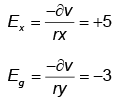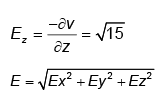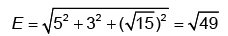E = 7

QUESTION: 17

Equipotential surfaces associated with an electric field which is increasing in magnitude along the x-direction are.

Solution:

Eequipotential planes will be normal to field, so they are in y-z plane.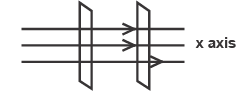QUESTION: 18

Figure shown three points A,B and C in a region of uniform electric field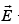The line AB is perpendicular and BC is parallel to the field lines. Then which of the following holds good. Where VA, VB and VC represent the electric potential at points A,B and C respectively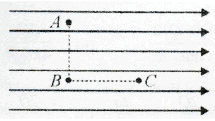Solution:

Electric field is always in direction of decreasing potential.
So VA = VB > VC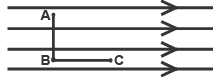QUESTION: 19

A charge +q is fixed at each of the points x = x0, x = 3x0, x = 5x0....... infinite, on the x-axis and a charge -q is fixed at each of the points x = 2x0, x = 4x0, x = 6x0..... infinite. Here x0 is a positive constant. Take the electric potential at a point due to a charge Q at a distance r from it to be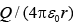. Then, the potential at the origin due to the above system of charges is

Solution: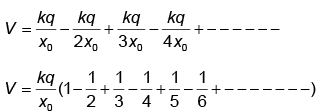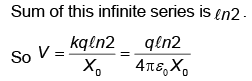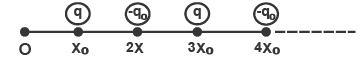QUESTION: 20

A non-conducting ring of radius 0.5 m carries a total charge of 1.11×10-10C distributed non-uniformly on its circumference producing an electric field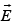everywhere in space. The value of the line integral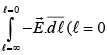being centre of the ring) in volts is

Solution:

Point to remembe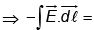negative of line intergral of electric field gives us the potential at a point.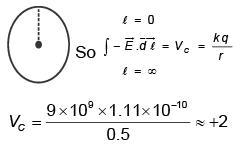QUESTION: 21

A charged particle q is shot towards another charged particle Q which is fixed, with a speed v. It approaches Q upto a closest distance r and then returns. if q were given a speed 2v, the closest distances of approach would be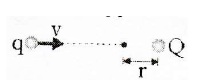Solution:

For fixed charge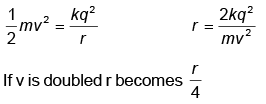QUESTION: 22

The electric potential at a point distant r from an electric dipole is proportional to

Solution: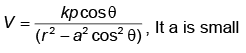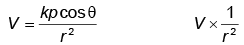QUESTION: 23

In the electric field of a point charge q shown, a charge is carried from A to B and from A to C. Compare the work done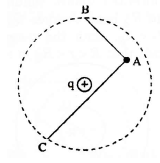Solution:

As circle is equi potential VB = VC so work done comes out to be same as ΔV = same.

QUESTION: 24

The potential at a point x (measured in µm) due to some charges situated on the X-axis is given byThe electric field E at x = 4 µm is given by :

Solution: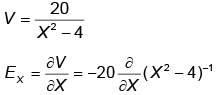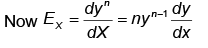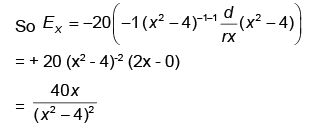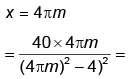QUESTION: 25

The electric potential at a point (x,y,z) is given by tential at a point (x,y,z) is given by V = x2 y - xz3 + 4
The electric fieldat that point is :

Solution: